# Data Visualisation - Histogram (Frequency distribution)

A histogram is a type of graph generally used to visualize a distribution

An histogram is also known as a frequency distribution.

Histograms can reveal information not captured by summary statistics such as:

of the distribution.

An histograms plots continuous data into bins (non-overlapping intervals)

For categorical data, you may want to see the bar charts.

A histogram is a combination of:

## Documentation / Reference

Discover More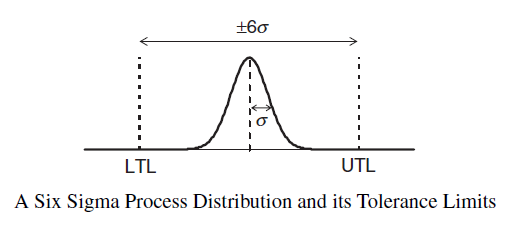Six Sigma (6s) is an approach to improve the performance of business process. where: sigma UTL = Upper Tolerance Limit. See LTL = Lower Tolerance Limit The 6s strategy was developed by Motorola,...D3 - Histogram

in D3. The properties of an histogram function A callback function to return the data to bins from the raw data (data passed to the histogram function). Parameters are: the element the index...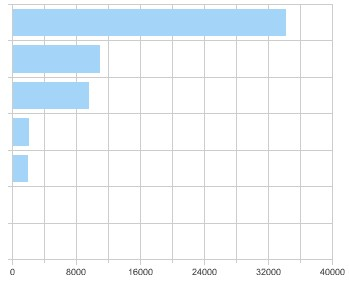Data Visualization - Bar Chart

Bar graphs plots: a categorical variable associated with quantities (number of case, sum of) histograms continuous variable associated with its bins They show: quantities as bar lengths...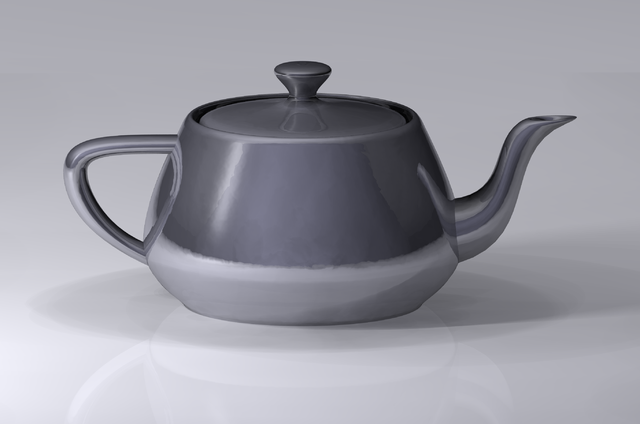Data Visualization Foundation

The Utah Teapot. Data visualization is the process of mapping quantitative data to visuals (shapes, color, position, etc) to create a graph made of geometric object. Information visualization: ...Frequency Distribution

A frequency distribution is a distribution of the frequency of each element ie the count of each element in a set or the count of each element in a period multiset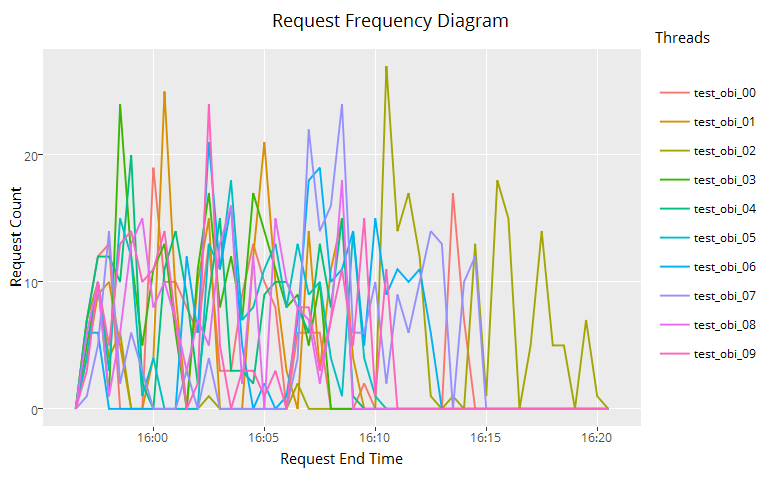Ggplot - Histogram (geom_histogram, geom_freqpoly)

geom_histogram = stat_bin + geom_bar + position = “stacked” geom_histogramgeom_freqpoly = stat_bin + geom_line + position = “identity” The below code handles outliers by: creating manually...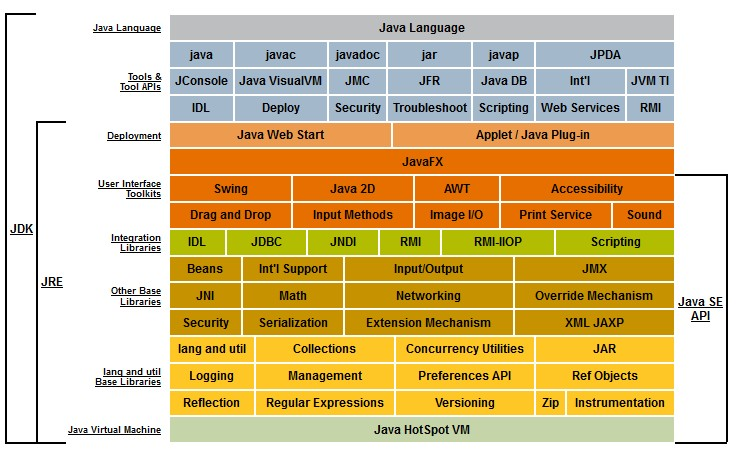Java - Map

Map is a data structure implementation of the java collection framework. See java/util/MapMap hashmap. Permits null values and the null key. (The HashMap class is roughly equivalent to Hashtable,...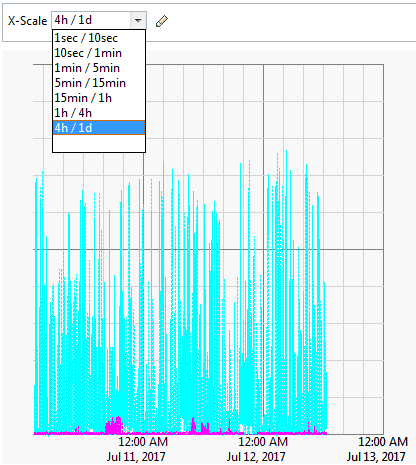Monitoring Metrics - Histogram

An Histogram is a statistical collector that ranks the measured values into predefined classes. It reports: a histogram the number of observations, mean, standard deviation, minimum...Oracle Database - Histogram Analytic

How to build an histogram in Oracle (and binning) You create equi-width (all bin have the same distance) histogram with the WIDTH_BUCKET function. where: column is a date or number column ...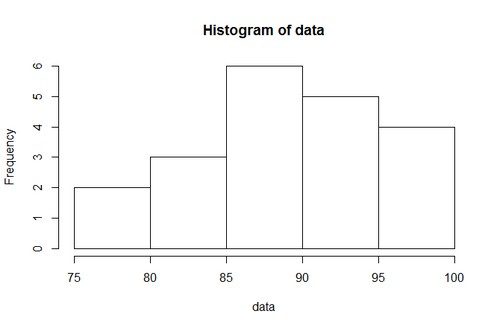R - Histogram

How to make histogram in R. plot“” You can use the layout function in order to have more than one histogram by page. Example: See : Histogram: Geom: bar Stat: bin Scale: linear...